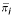Effect Sizep Noether = Pr(Y >X)Sample size formulae1. Δ Normal is the standardised effect size index, μ T and μ C are the expected group means of outcome variable under the null and alternative hypotheses and σ is the standard deviation of outcome variable (assumed the same under the null and alternative hypotheses). pNoether is an estimate of the probability that an observation drawn at random from population Y would exceed an observation drawn at random from population X. Let π iT be the probability of being in category i in Group T and γ iT be the expected cumulative probability of being in category i or less in Group T (i.e. γ iT = Pr(Y ≤ yi)).is the combined mean (of the proportion of patients expected in groups T and C) for each category i. z1-α/2and z1-β are the appropriate values from the standard Normal distribution for the 100 (1 - α/2) and 100 (1 - β) percentiles respectively. Number of subjects per group n for a two-sided significance level α and power 1 - β.